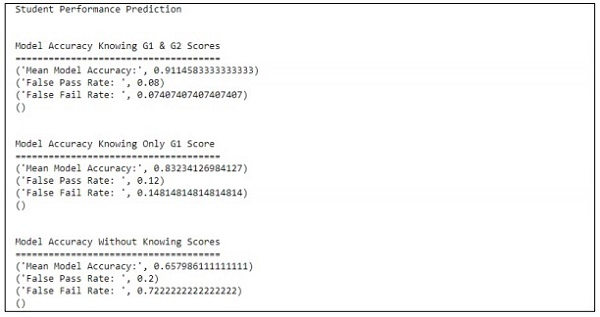# Improving Prediction Performance

In this chapter, we will focus on building a model that helps in the prediction of student’s performance with a number of attributes included in it. The focus is to display the failure result of students in an examination.

## Process

The target value of assessment is G3. This values can be binned and further classified as failure and success. If G3 value is greater than or equal to 10, then the student passes the examination.

## Example

Consider the following example wherein a code is executed to predict the performance if students −

```import pandas as pd
""" Read data file as DataFrame """
""" Import ML helpers """
from sklearn.preprocessing import LabelEncoder
from sklearn.model_selection import train_test_split
from sklearn.metrics import confusion_matrix
from sklearn.model_selection import GridSearchCV, cross_val_score
from sklearn.pipeline import Pipeline
from sklearn.feature_selection import SelectKBest, chi2
from sklearn.svm import LinearSVC # Support Vector Machine Classifier model
""" Split Data into Training and Testing Sets """
def split_data(X, Y):
return train_test_split(X, Y, test_size=0.2, random_state=17)
""" Confusion Matrix """
def confuse(y_true, y_pred):
cm = confusion_matrix(y_true=y_true, y_pred=y_pred)
# print("\nConfusion Matrix: \n", cm)
fpr(cm)
ffr(cm)
""" False Pass Rate """
def fpr(confusion_matrix):
fp = confusion_matrix
tf = confusion_matrix
rate = float(fp) / (fp + tf)
print("False Pass Rate: ", rate)
""" False Fail Rate """
def ffr(confusion_matrix):
ff = confusion_matrix
tp = confusion_matrix
rate = float(ff) / (ff + tp)
print("False Fail Rate: ", rate)
return rate
""" Train Model and Print Score """
def train_and_score(X, y):
X_train, X_test, y_train, y_test = split_data(X, y)
clf = Pipeline([
('reduce_dim', SelectKBest(chi2, k=2)),
('train', LinearSVC(C=100))
])
scores = cross_val_score(clf, X_train, y_train, cv=5, n_jobs=2)
print("Mean Model Accuracy:", np.array(scores).mean())
clf.fit(X_train, y_train)
confuse(y_test, clf.predict(X_test))
print()
""" Main Program """
def main():
print("\nStudent Performance Prediction")
# For each feature, encode to categorical values
class_le = LabelEncoder()
for column in df[["school", "sex", "address", "famsize", "Pstatus", "Mjob",
"Fjob", "reason", "guardian", "schoolsup", "famsup", "paid", "activities",
"nursery", "higher", "internet", "romantic"]].columns:
df[column] = class_le.fit_transform(df[column].values)
# Encode G1, G2, G3 as pass or fail binary values
for i, row in df.iterrows():
if row["G1"] >= 10:
df["G1"][i] = 1
else:
df["G1"][i] = 0
if row["G2"] >= 10:
df["G2"][i] = 1
else:
df["G2"][i] = 0
if row["G3"] >= 10:
df["G3"][i] = 1
else:
df["G3"][i] = 0
# Target values are G3
y = df.pop("G3")
# Feature set is remaining features
X = df
print("\n\nModel Accuracy Knowing G1 & G2 Scores")
print("=====================================")
train_and_score(X, y)
X.drop(["G2"], axis = 1, inplace=True)
print("\n\nModel Accuracy Knowing Only G1 Score")
print("=====================================")
train_and_score(X, y)
X.drop(["G1"], axis=1, inplace=True)
print("\n\nModel Accuracy Without Knowing Scores")
print("=====================================")
train_and_score(X, y)
main()
```

## Output

The above code generates the output as shown below

The prediction is treated with reference to only one variable. With reference to one variable, the student performance prediction is as shown below −